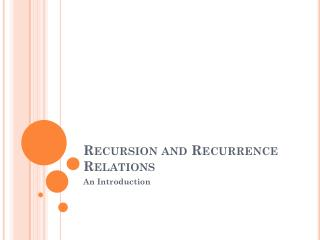DownloadDownload PresentationRecursion and Recurrence Relations

# Recursion and Recurrence Relations

Télécharger la présentation## Recursion and Recurrence Relations

- - - - - - - - - - - - - - - - - - - - - - - - - - - E N D - - - - - - - - - - - - - - - - - - - - - - - - - - -
##### Presentation Transcript

1. Recursion and Recurrence Relations An Introduction

2. Recursion • A technique of defining a function, a set or an algorithm in terms of itself is a “recursion”. • For Example: • Factorial !n=n X !(n-1), and !0=!1=1 • Fibonacci sequence f0 =1, f1 =1 and fk =fk-2 +fk-1 for k≥2

3. Solution of a problem with recursion and Iteration • Lets solve this problem with to methods • Recursion • Iteration Telescoping form of a polynomial expression Eg. X5 + 3x4 – 15x2 +x -10 Its telescoping form is (((x((x)+3)))x-15)x+1)x-10

4. Types of Recurrence Relation • Linear Recurrence relation with constant coefficients. c0ar + c1 ar-1 + c2 ar-2 + ……..+ ckar-k =f(r) • Homogeneous recurrence Relation s(k)+c1 s(k-1) +c2 s(k-2)+…….+ cn s(k-n)=f(k) and f(n)=0 • Non-Homogeneous recurrence Relation • s(k)+c1 s(k-1) +c2 s(k-2)+…….+ cn s(k-n)=f(k) and f(n)=non-zero.